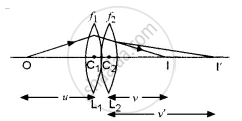# When Two Thin Lenses of Focal Lengths F1 and F2 Are Kept Co Axially and in Contact, Prove that Their Combined Focal Length ‘F’ is Given By: - Physics (Theory)

Numerical

When two thin lenses of focal lengths f1 and f2 are kept coaxially and in contact, prove that their combined focal length ‘f’ is given by: 1/f = 1/f_1 + 1/f_2

#### Solution

Let two thin lenses L1 and L2 of focal lengths f1 and f2 be put in contact. O is a point object at a distance u from the lens L1 Its image is formed at I after refraction through the two lenses at a distance v from the combination. The lens L1 forms the image of O at I’. I’, then serves as a virtual object for the lens L2 which forms a real image at I.Now, we know that by lens formula
1/v - 1/u = 1/f

Applying this relation for refraction at the lens
L1 of focal length f1 can be written as

1/v' - 1/u = 1/f_1    ...(i)

For refraction at the lens L2
u = v', v = v

∴ For this lens 1/v - 1/v' = 1/f_2    ...(ii)

And if F is the focal length of the combination, then

1/v - 1/u = 1/F

Adding (i) and (ii), we get

1/v' - 1/u + 1/v - 1/v' = 1/f_1  + 1/f_2

or 1/v -1/u = 1/f_1  + 1/f_2

or 1/"F" = 1/f_1  + 1/f_2

Concept: Lenses
Is there an error in this question or solution?